Скачать презентацию Discrete optimization methods in computer vision Nikos Komodakis

4129995a33a1d29667a561ee23046b3c.ppt

• Количество слайдов: 55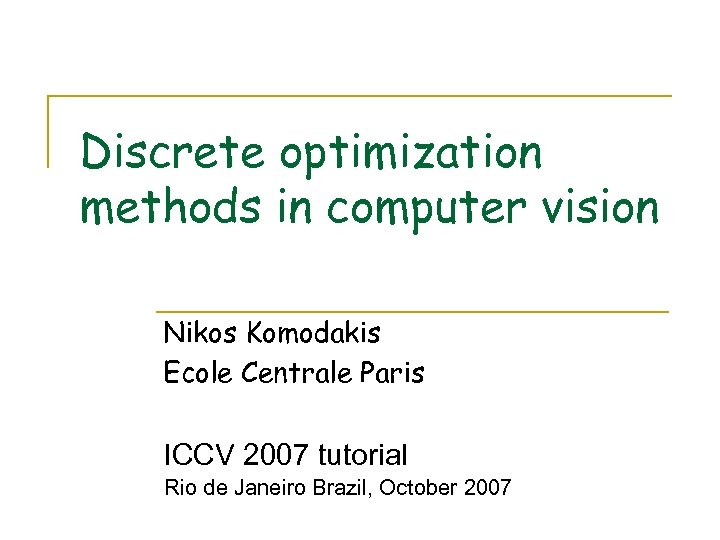Discrete optimization methods in computer vision Nikos Komodakis Ecole Centrale Paris ICCV 2007 tutorial Rio de Janeiro Brazil, October 2007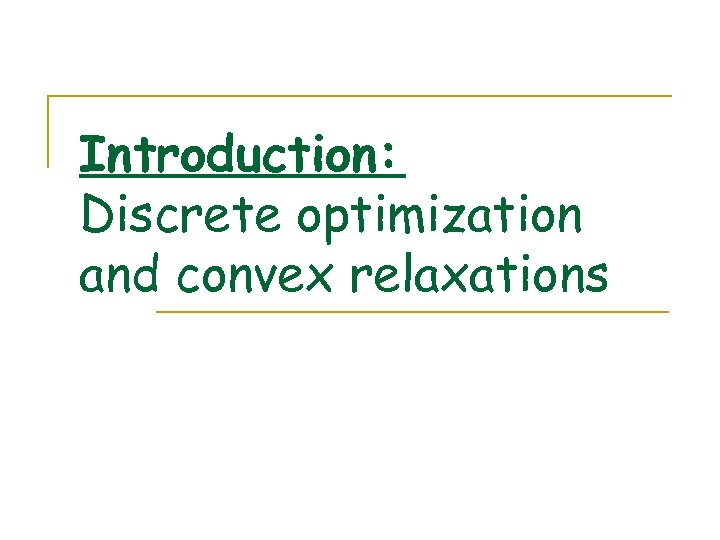Introduction: Discrete optimization and convex relaxations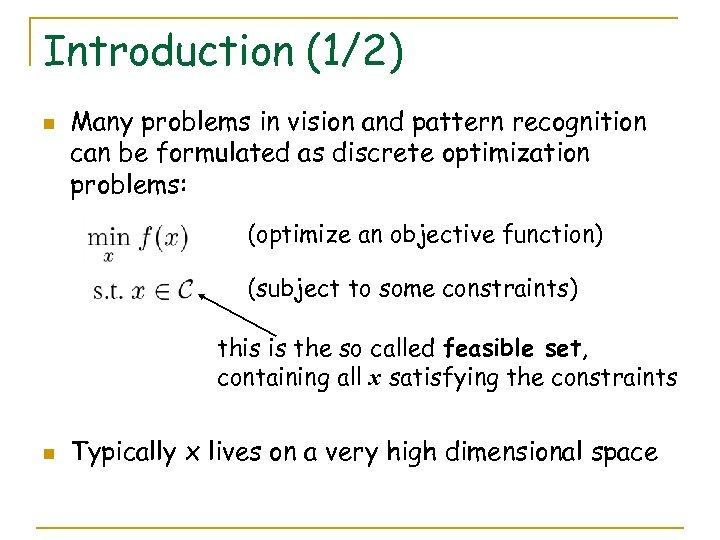Introduction (1/2) n Many problems in vision and pattern recognition can be formulated as discrete optimization problems: (optimize an objective function) (subject to some constraints) this is the so called feasible set, containing all x satisfying the constraints n Typically x lives on a very high dimensional space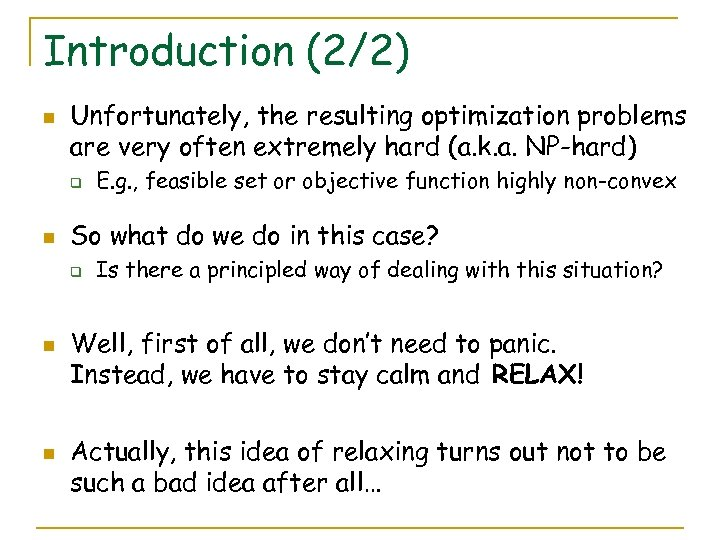Introduction (2/2) n Unfortunately, the resulting optimization problems are very often extremely hard (a. k. a. NP-hard) q n So what do we do in this case? q n n E. g. , feasible set or objective function highly non-convex Is there a principled way of dealing with this situation? Well, first of all, we don’t need to panic. Instead, we have to stay calm and RELAX! Actually, this idea of relaxing turns out not to be such a bad idea after all…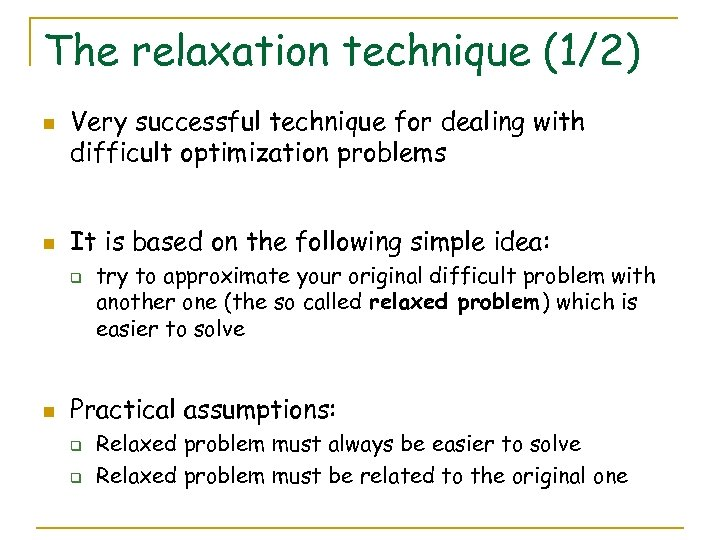The relaxation technique (1/2) n n Very successful technique for dealing with difficult optimization problems It is based on the following simple idea: q n try to approximate your original difficult problem with another one (the so called relaxed problem) which is easier to solve Practical assumptions: q q Relaxed problem must always be easier to solve Relaxed problem must be related to the original one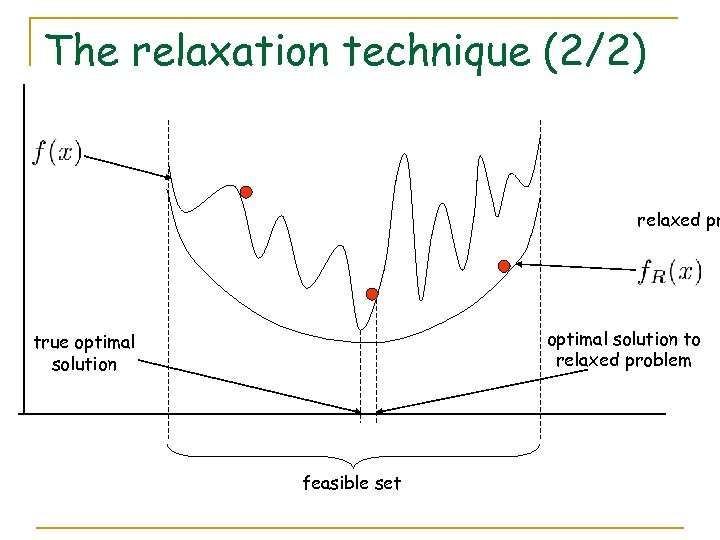The relaxation technique (2/2) relaxed pr optimal solution to relaxed problem true optimal solution feasible set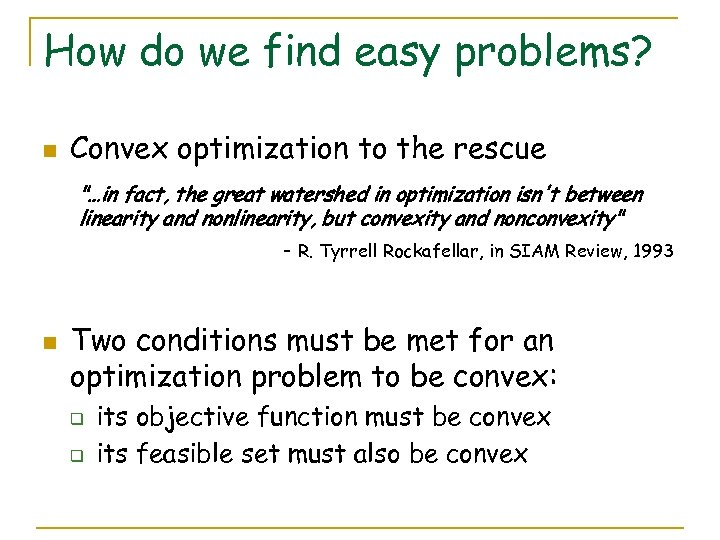How do we find easy problems? n Convex optimization to the rescue "…in fact, the great watershed in optimization isn't between linearity and nonlinearity, but convexity and nonconvexity" - R. Tyrrell Rockafellar, in SIAM Review, 1993 n Two conditions must be met for an optimization problem to be convex: q q its objective function must be convex its feasible set must also be convex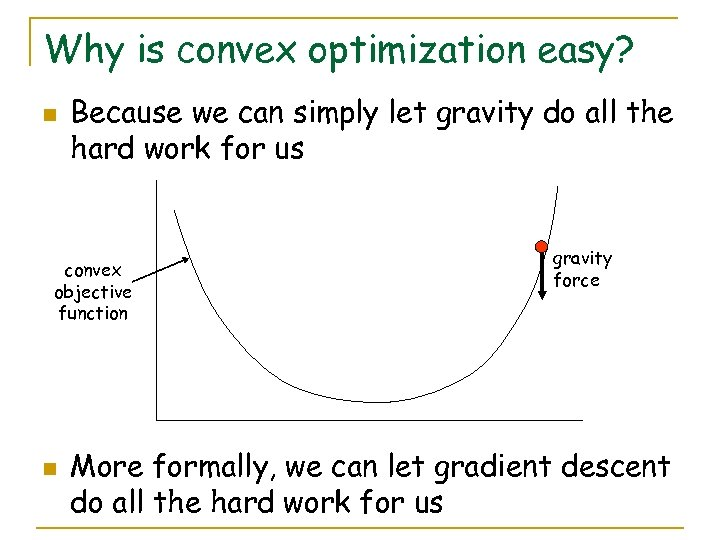Why is convex optimization easy? n Because we can simply let gravity do all the hard work for us convex objective function n gravity force More formally, we can let gradient descent do all the hard work for us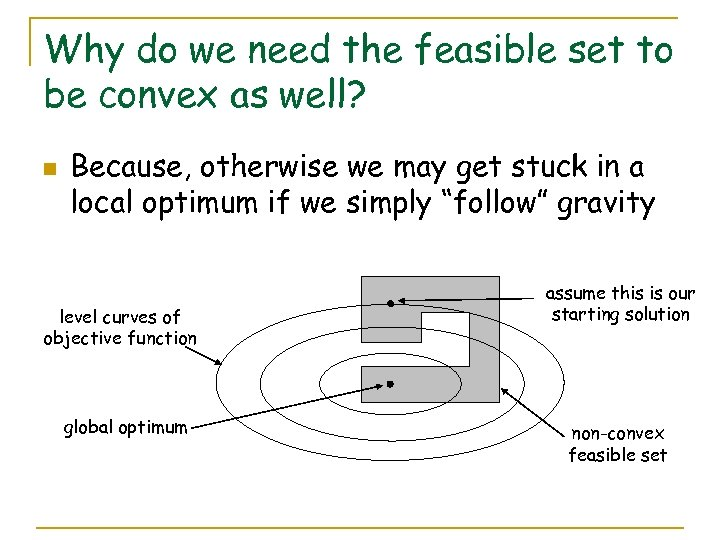Why do we need the feasible set to be convex as well? n Because, otherwise we may get stuck in a local optimum if we simply “follow” gravity level curves of objective function global optimum assume this is our starting solution non-convex feasible set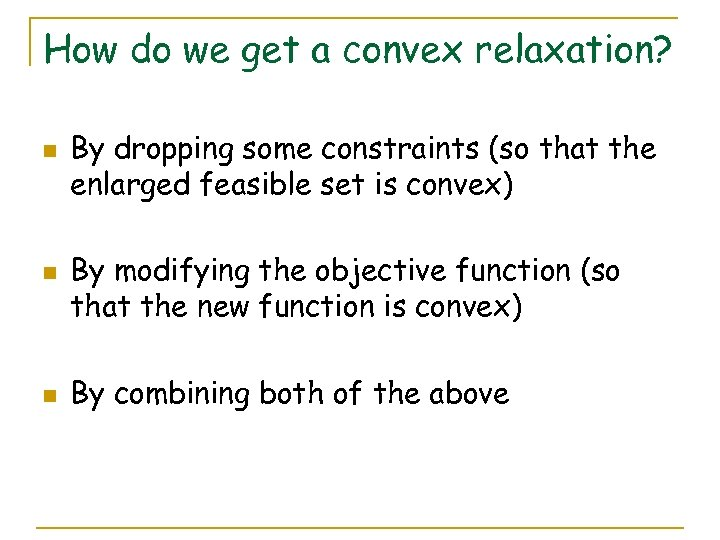How do we get a convex relaxation? n n n By dropping some constraints (so that the enlarged feasible set is convex) By modifying the objective function (so that the new function is convex) By combining both of the above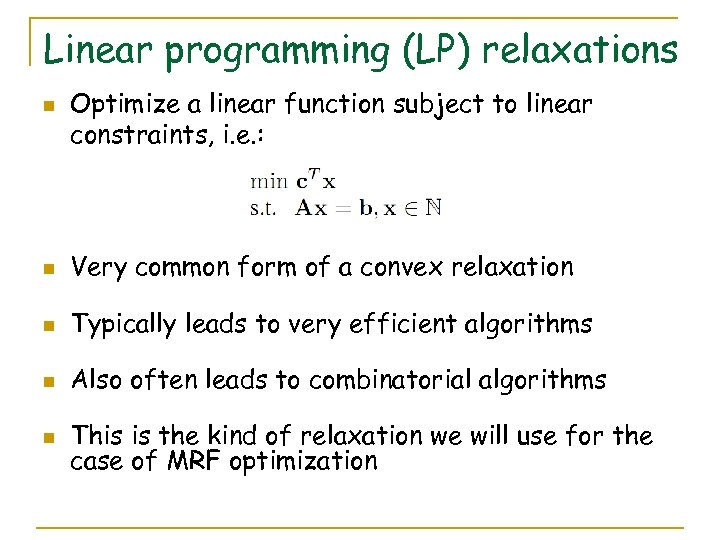Linear programming (LP) relaxations n Optimize a linear function subject to linear constraints, i. e. : n Very common form of a convex relaxation n Typically leads to very efficient algorithms n Also often leads to combinatorial algorithms n This is the kind of relaxation we will use for the case of MRF optimization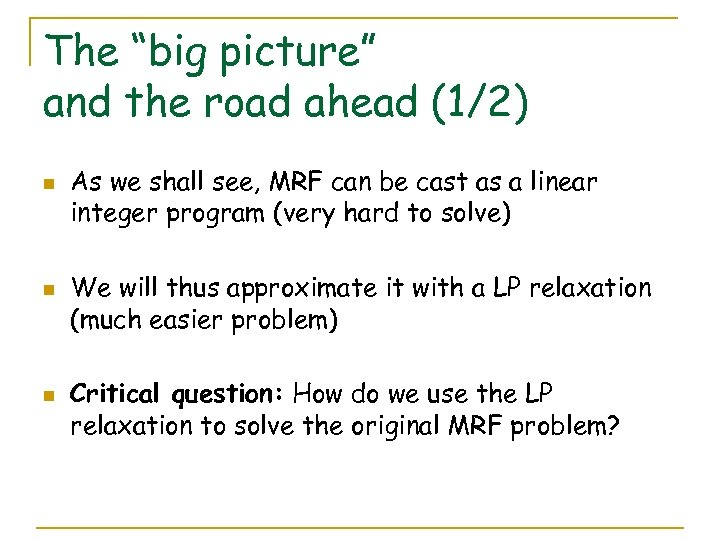The “big picture” and the road ahead (1/2) n n n As we shall see, MRF can be cast as a linear integer program (very hard to solve) We will thus approximate it with a LP relaxation (much easier problem) Critical question: How do we use the LP relaxation to solve the original MRF problem?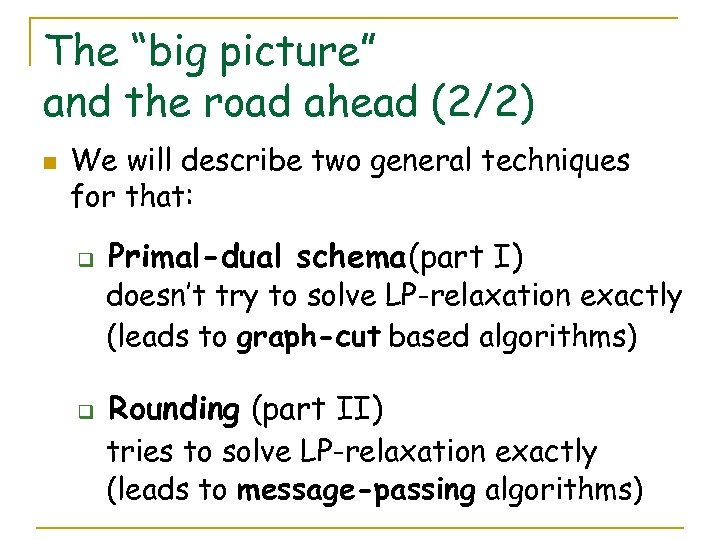The “big picture” and the road ahead (2/2) n We will describe two general techniques for that: q Primal-dual schema (part I) doesn’t try to solve LP-relaxation exactly (leads to graph-cut based algorithms) q Rounding (part II) tries to solve LP-relaxation exactly (leads to message-passing algorithms)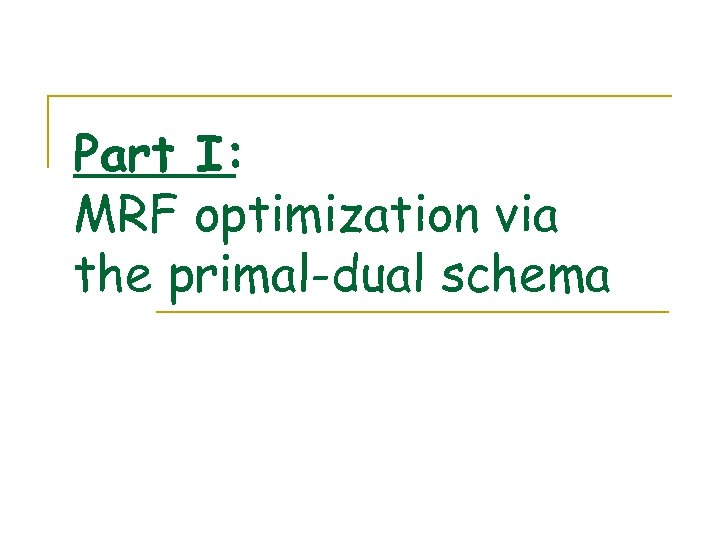Part I: MRF optimization via the primal-dual schema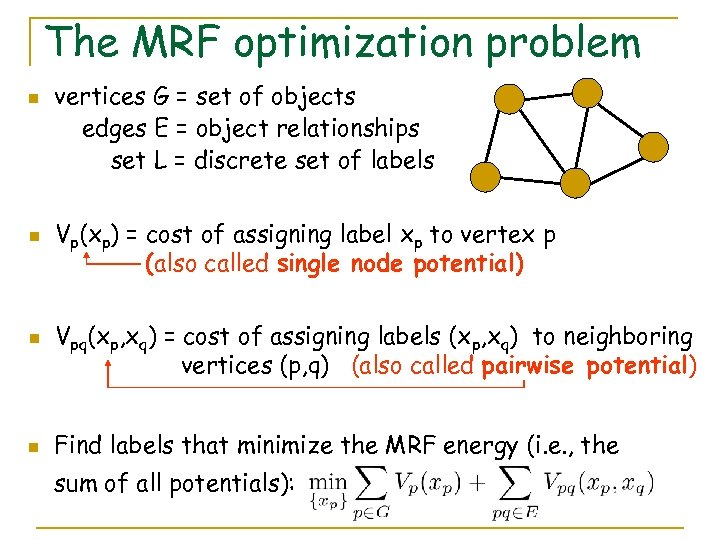The MRF optimization problem n n vertices G = set of objects edges E = object relationships set L = discrete set of labels Vp(xp) = cost of assigning label xp to vertex p (also called single node potential) Vpq(xp, xq) = cost of assigning labels (xp, xq) to neighboring vertices (p, q) (also called pairwise potential) Find labels that minimize the MRF energy (i. e. , the sum of all potentials):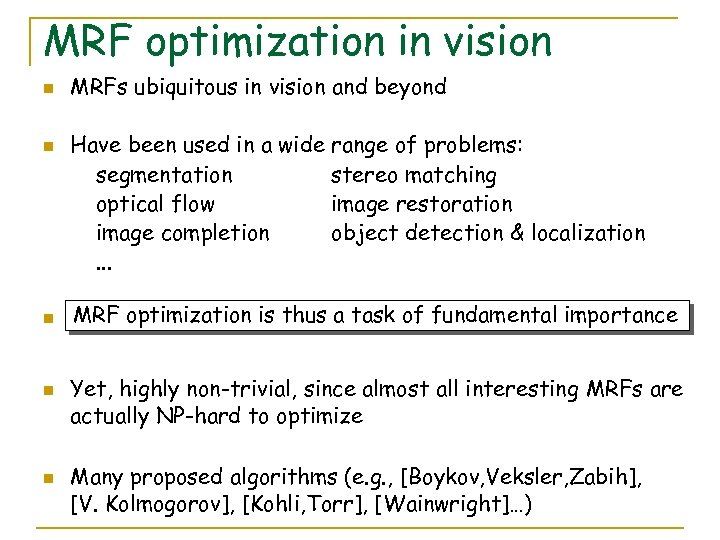MRF optimization in vision n n MRFs ubiquitous in vision and beyond Have been used in a wide range of problems: segmentation stereo matching optical flow image restoration image completion object detection & localization. . . MRF optimization is thus a task of fundamental importance Yet, highly non-trivial, since almost all interesting MRFs are actually NP-hard to optimize Many proposed algorithms (e. g. , [Boykov, Veksler, Zabih], [V. Kolmogorov], [Kohli, Torr], [Wainwright]…)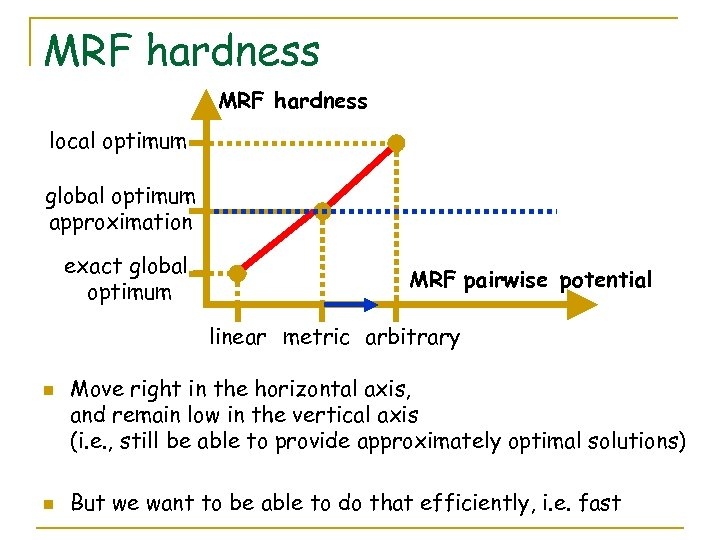MRF hardness local optimum global optimum approximation exact global optimum MRF pairwise potential linear metric arbitrary n n Move right in the horizontal axis, and remain low in the vertical axis (i. e. , still be able to provide approximately optimal solutions) But we want to be able to do that efficiently, i. e. fast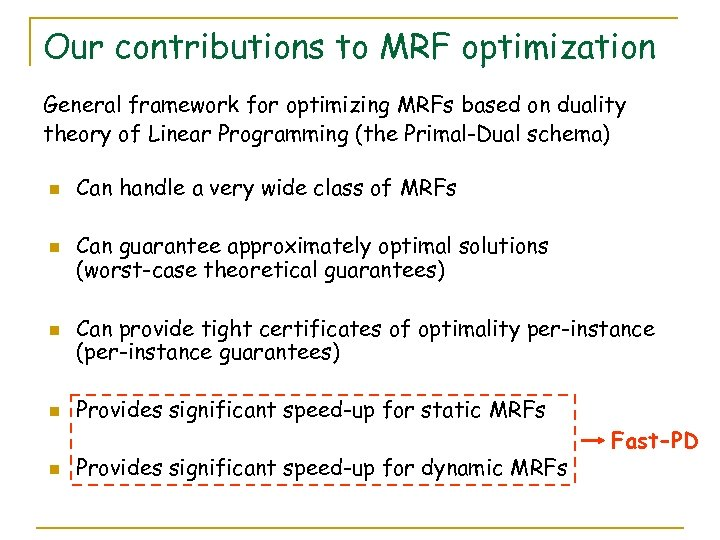Our contributions to MRF optimization General framework for optimizing MRFs based on duality theory of Linear Programming (the Primal-Dual schema) n n n Can handle a very wide class of MRFs Can guarantee approximately optimal solutions (worst-case theoretical guarantees) Can provide tight certificates of optimality per-instance (per-instance guarantees) Provides significant speed-up for static MRFs Provides significant speed-up for dynamic MRFs Fast-PD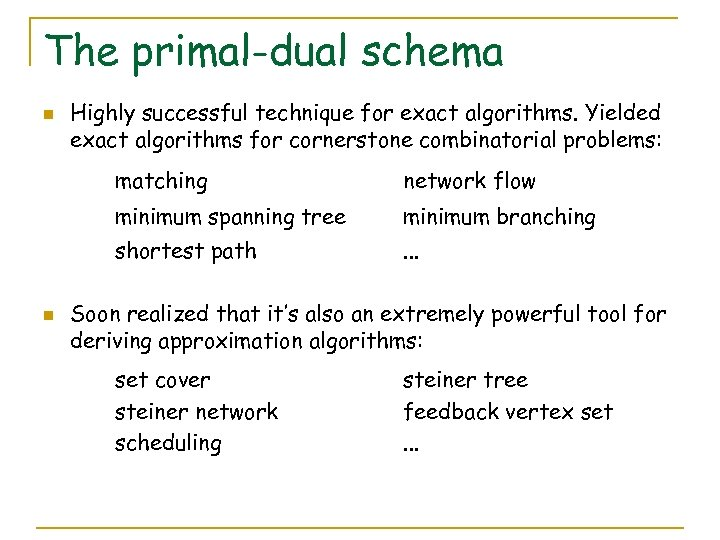The primal-dual schema n Highly successful technique for exact algorithms. Yielded exact algorithms for cornerstone combinatorial problems: matching minimum spanning tree minimum branching shortest path n network flow. . . Soon realized that it’s also an extremely powerful tool for deriving approximation algorithms: set cover steiner network scheduling steiner tree feedback vertex set. . .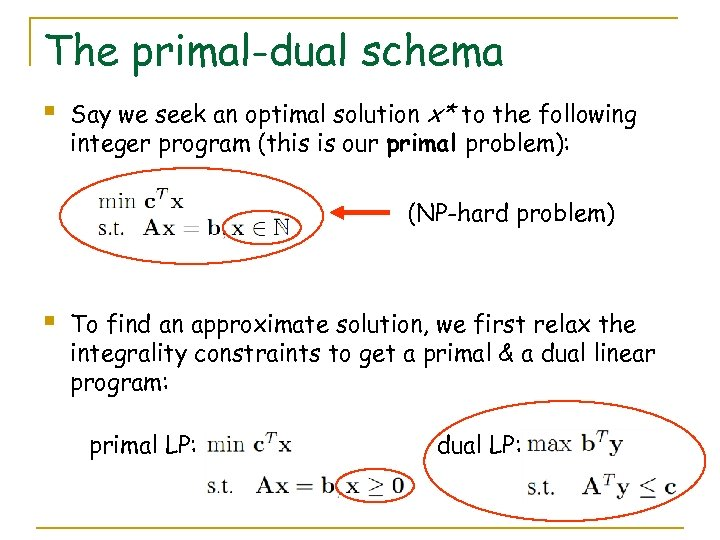The primal-dual schema § Say we seek an optimal solution x* to the following integer program (this is our primal problem): (NP-hard problem) § To find an approximate solution, we first relax the integrality constraints to get a primal & a dual linear program: primal LP: dual LP: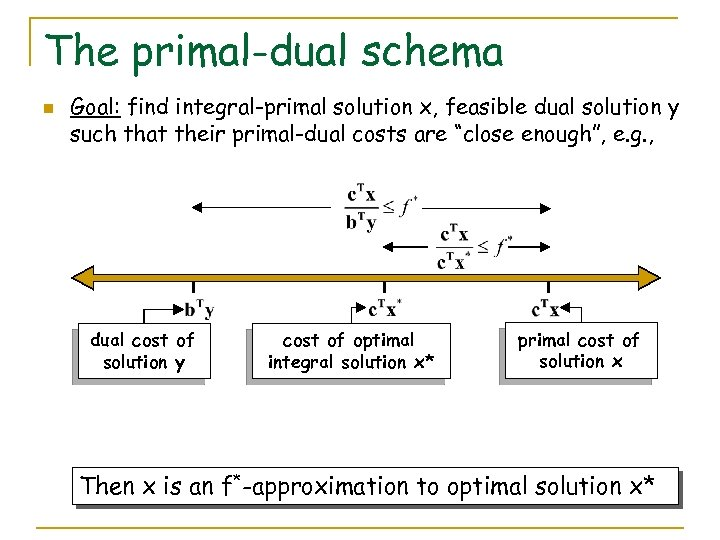The primal-dual schema n Goal: find integral-primal solution x, feasible dual solution y such that their primal-dual costs are “close enough”, e. g. , dual cost of solution y cost of optimal integral solution x* primal cost of solution x Then x is an f*-approximation to optimal solution x*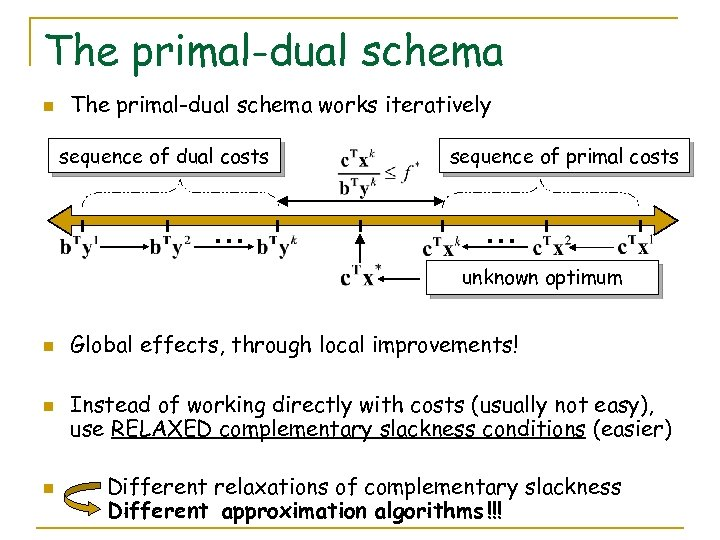The primal-dual schema n The primal-dual schema works iteratively sequence of dual costs … sequence of primal costs … unknown optimum n n n Global effects, through local improvements! Instead of working directly with costs (usually not easy), use RELAXED complementary slackness conditions (easier) Different relaxations of complementary slackness Different approximation algorithms !!!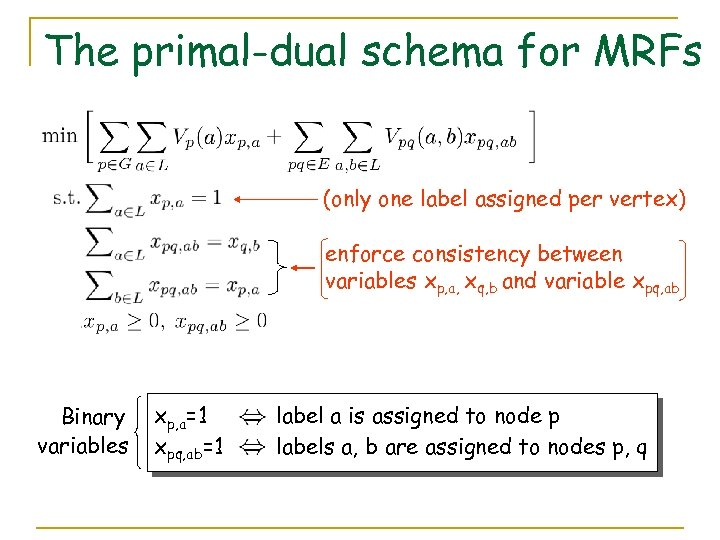The primal-dual schema for MRFs (only one label assigned per vertex) enforce consistency between variables xp, a, xq, b and variable xpq, ab Binary variables xp, a=1 xpq, ab=1 label a is assigned to node p labels a, b are assigned to nodes p, q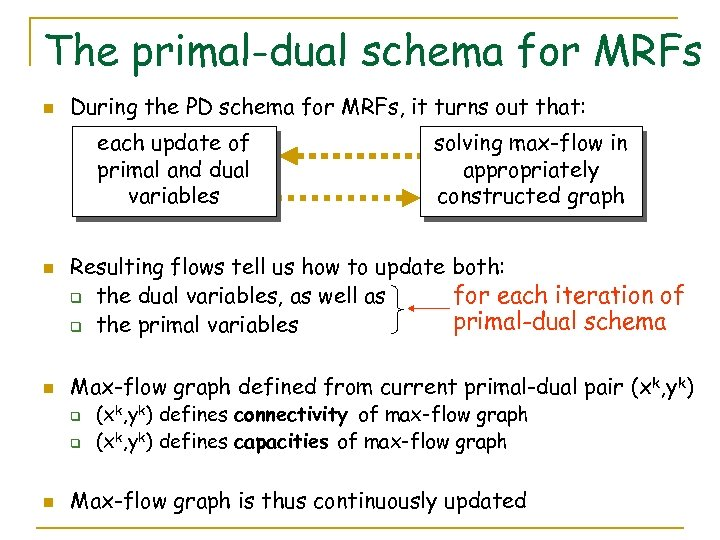The primal-dual schema for MRFs n During the PD schema for MRFs, it turns out that: each update of primal and dual variables n n Resulting flows tell us how to update both: for each iteration of q the dual variables, as well as primal-dual schema q the primal variables Max-flow graph defined from current primal-dual pair (xk, yk) q q n solving max-flow in appropriately constructed graph (xk, yk) defines connectivity of max-flow graph (xk, yk) defines capacities of max-flow graph Max-flow graph is thus continuously updated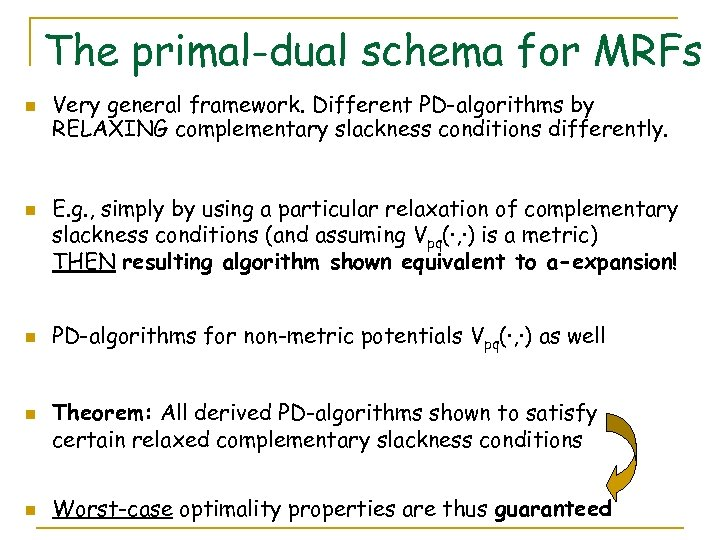The primal-dual schema for MRFs n n n Very general framework. Different PD-algorithms by RELAXING complementary slackness conditions differently. E. g. , simply by using a particular relaxation of complementary slackness conditions (and assuming Vpq(·, ·) is a metric) THEN resulting algorithm shown equivalent to a-expansion! PD-algorithms for non-metric potentials Vpq(·, ·) as well Theorem: All derived PD-algorithms shown to satisfy certain relaxed complementary slackness conditions Worst-case optimality properties are thus guaranteed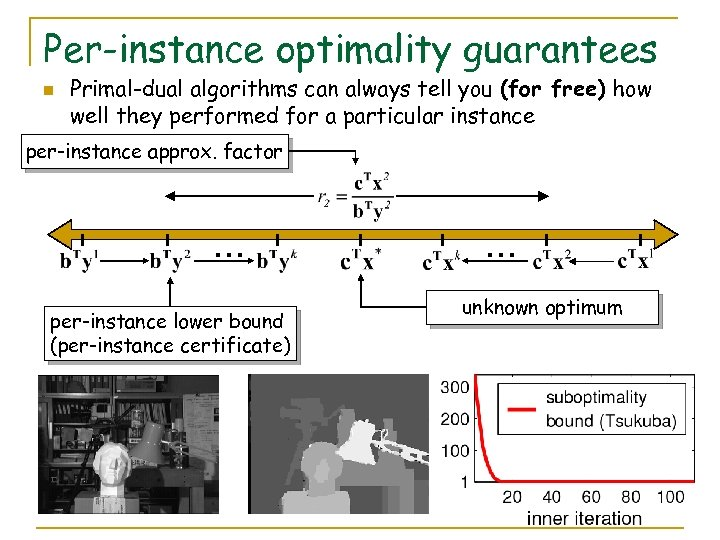Per-instance optimality guarantees n Primal-dual algorithms can always tell you (for free) how well they performed for a particular instance per-instance approx. factor … per-instance lower bound (per-instance certificate) … unknown optimum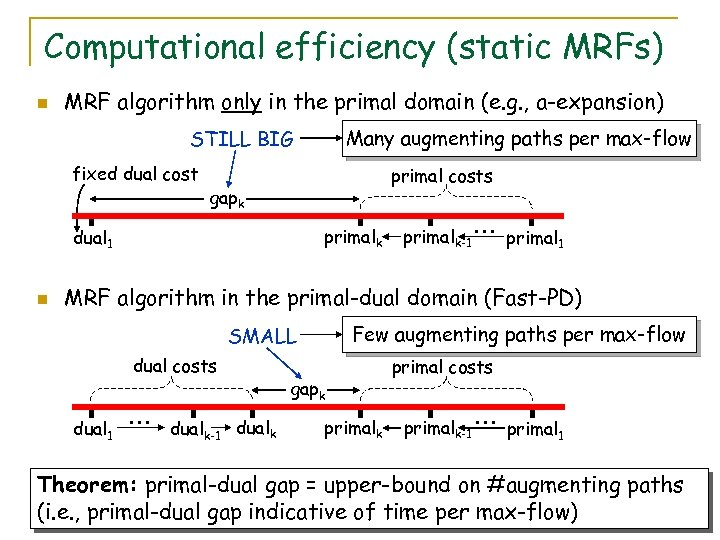Computational efficiency (static MRFs) n MRF algorithm only in the primal domain (e. g. , a-expansion) Many augmenting paths per max-flow STILL BIG fixed dual cost primal costs gapk primalk dual 1 n primalk-1 … primal 1 MRF algorithm in the primal-dual domain (Fast-PD) Few augmenting paths per max-flow SMALL dual costs dual 1 … dualk-1 dualk gapk primal costs primalk-1 … primal 1 Theorem: primal-dual gap = upper-bound on #augmenting paths (i. e. , primal-dual gap indicative of time per max-flow)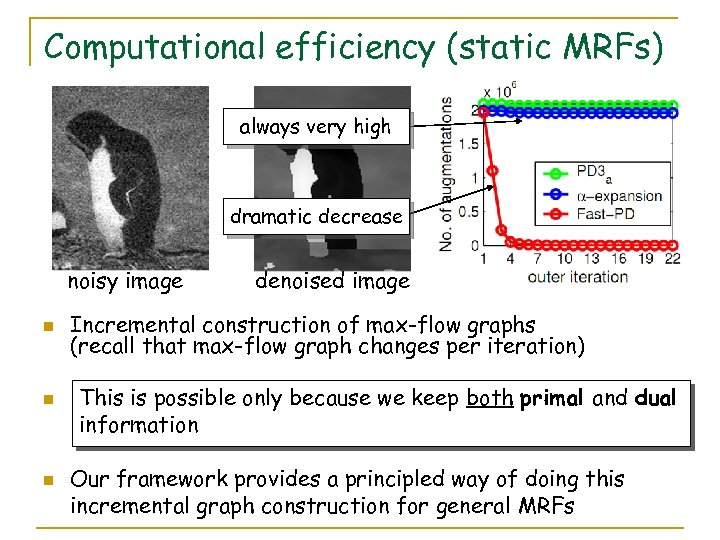Computational efficiency (static MRFs) always very high dramatic decrease noisy image n n n denoised image Incremental construction of max-flow graphs (recall that max-flow graph changes per iteration) This is possible only because we keep both primal and dual information Our framework provides a principled way of doing this incremental graph construction for general MRFs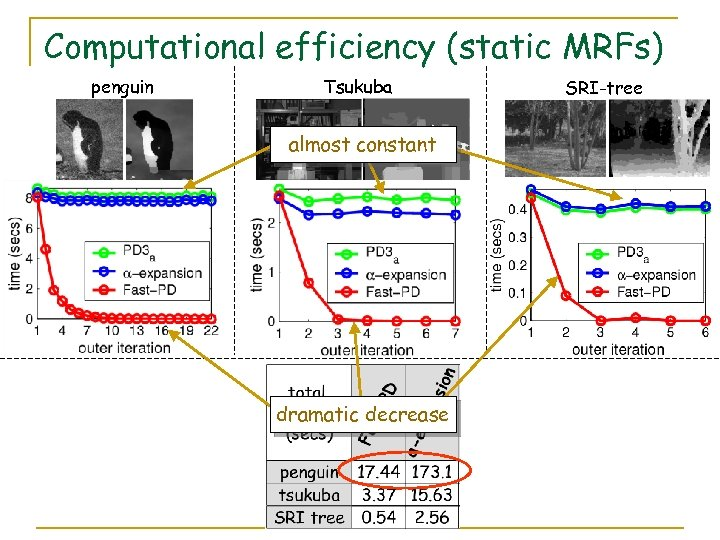Computational efficiency (static MRFs) penguin Tsukuba almost constant dramatic decrease SRI-tree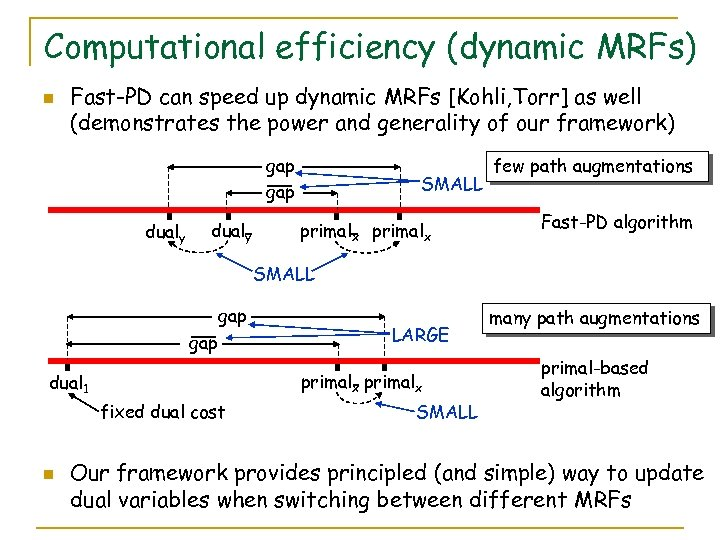Computational efficiency (dynamic MRFs) n Fast-PD can speed up dynamic MRFs [Kohli, Torr] as well (demonstrates the power and generality of our framework) gap dualy SMALL primalx few path augmentations Fast-PD algorithm SMALL gap primalx dual 1 fixed dual cost n LARGE SMALL many path augmentations primal-based algorithm Our framework provides principled (and simple) way to update dual variables when switching between different MRFs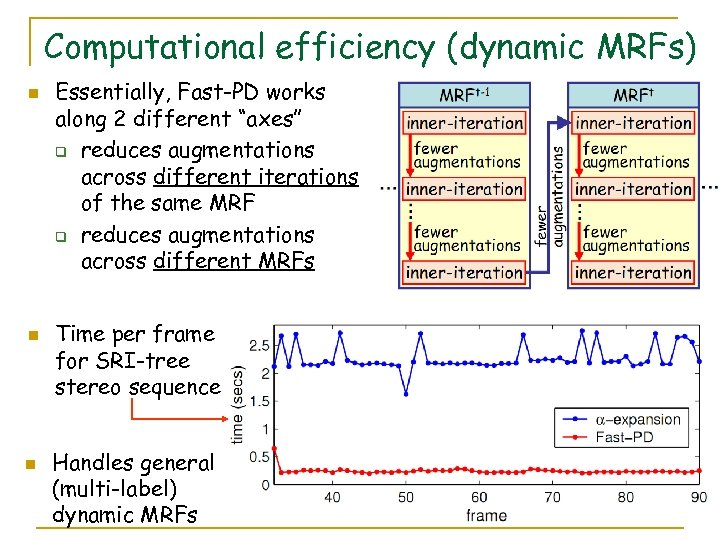Computational efficiency (dynamic MRFs) n n n Essentially, Fast-PD works along 2 different “axes” q reduces augmentations across different iterations of the same MRF q reduces augmentations across different MRFs Time per frame for SRI-tree stereo sequence Handles general (multi-label) dynamic MRFs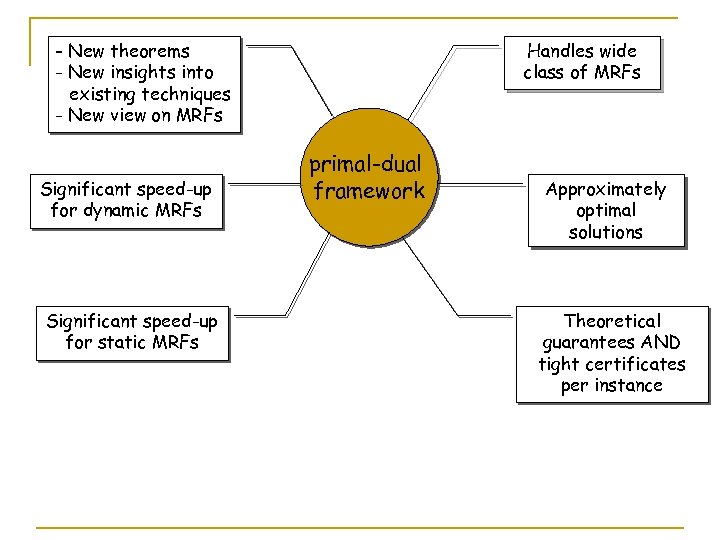- New theorems - New insights into existing techniques - New view on MRFs Significant speed-up for dynamic MRFs Significant speed-up for static MRFs Handles wide class of MRFs primal-dual framework Approximately optimal solutions Theoretical guarantees AND tight certificates per instance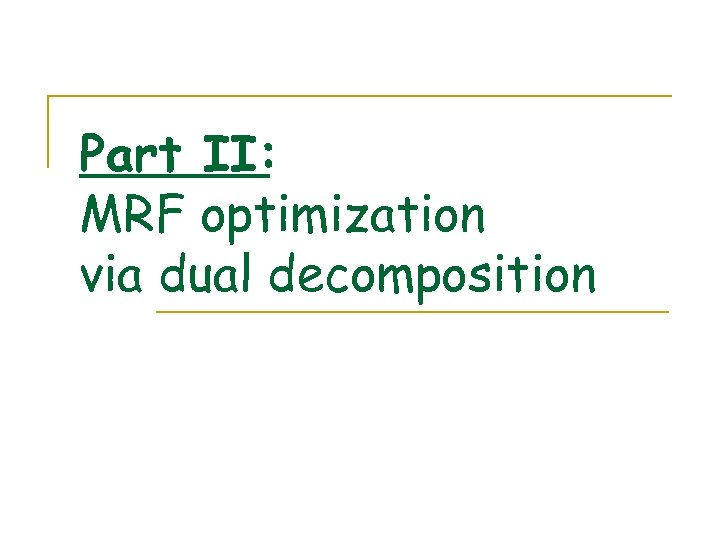Part II: MRF optimization via dual decomposition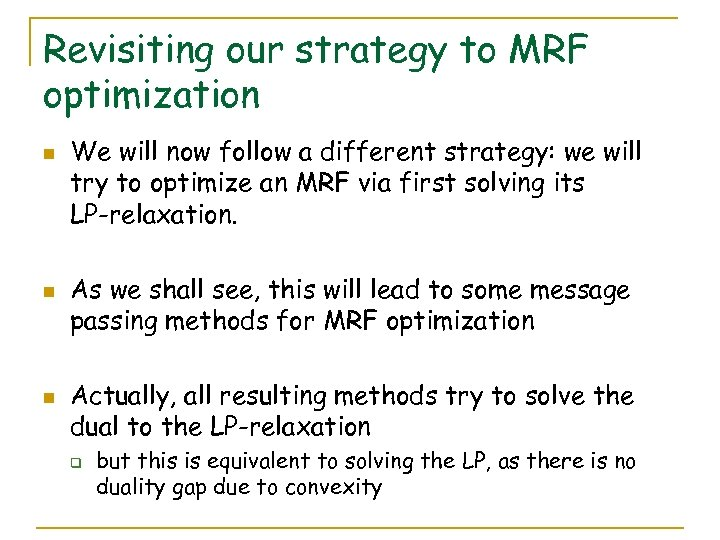Revisiting our strategy to MRF optimization n We will now follow a different strategy: we will try to optimize an MRF via first solving its LP-relaxation. As we shall see, this will lead to some message passing methods for MRF optimization Actually, all resulting methods try to solve the dual to the LP-relaxation q but this is equivalent to solving the LP, as there is no duality gap due to convexity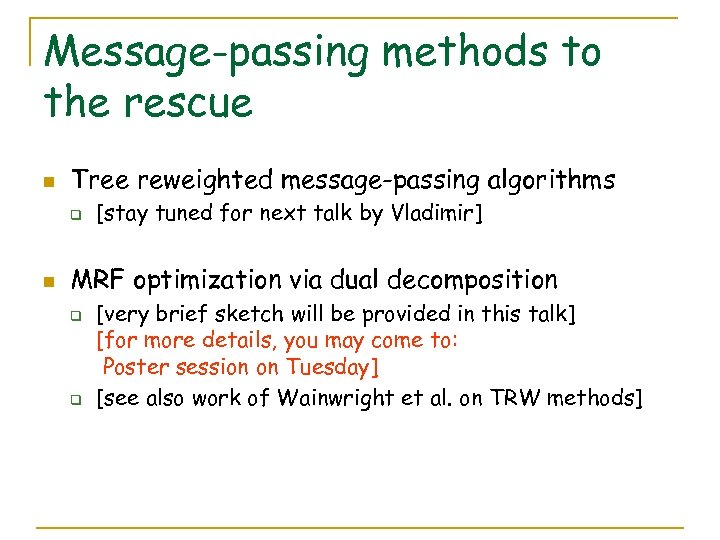Message-passing methods to the rescue n Tree reweighted message-passing algorithms q n [stay tuned for next talk by Vladimir] MRF optimization via dual decomposition q q [very brief sketch will be provided in this talk] [for more details, you may come to: Poster session on Tuesday] [see also work of Wainwright et al. on TRW methods]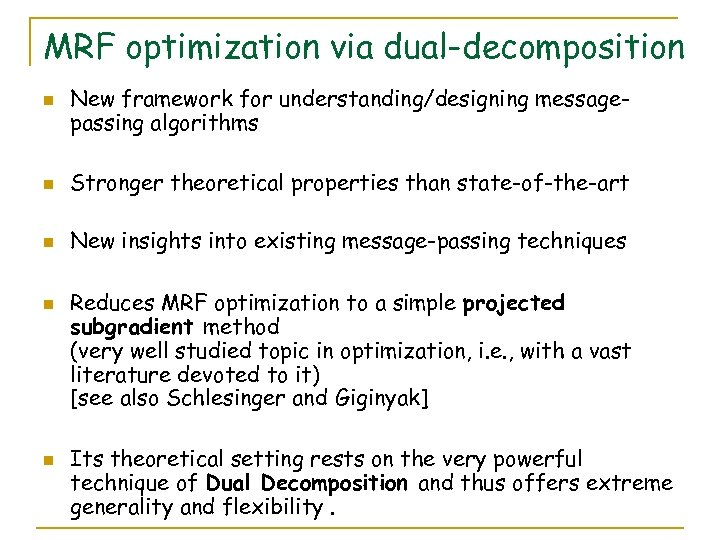MRF optimization via dual-decomposition n New framework for understanding/designing messagepassing algorithms n Stronger theoretical properties than state-of-the-art n New insights into existing message-passing techniques n n Reduces MRF optimization to a simple projected subgradient method (very well studied topic in optimization, i. e. , with a vast literature devoted to it) [see also Schlesinger and Giginyak] Its theoretical setting rests on the very powerful technique of Dual Decomposition and thus offers extreme generality and flexibility.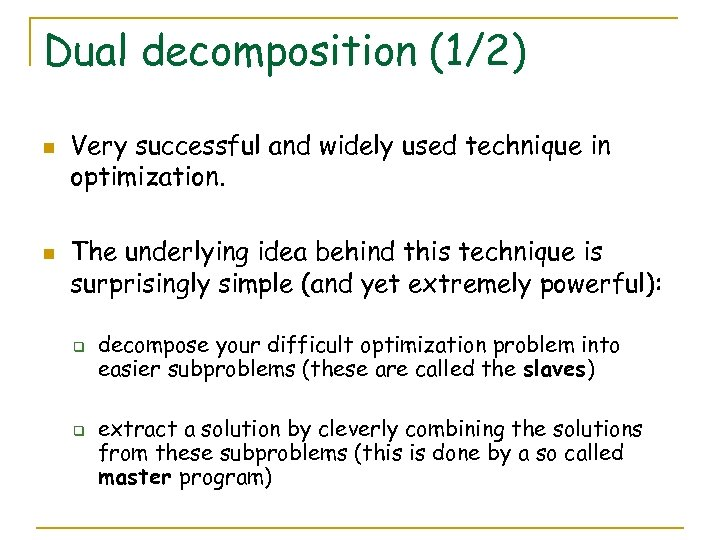Dual decomposition (1/2) n n Very successful and widely used technique in optimization. The underlying idea behind this technique is surprisingly simple (and yet extremely powerful): q q decompose your difficult optimization problem into easier subproblems (these are called the slaves) extract a solution by cleverly combining the solutions from these subproblems (this is done by a so called master program)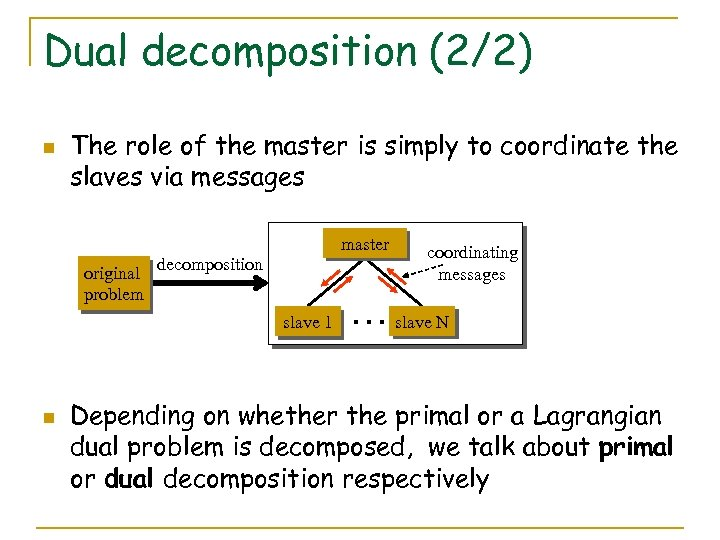Dual decomposition (2/2) n The role of the master is simply to coordinate the slaves via messages master original decomposition problem slave 1 n coordinating messages … slave N Depending on whether the primal or a Lagrangian dual problem is decomposed, we talk about primal or dual decomposition respectively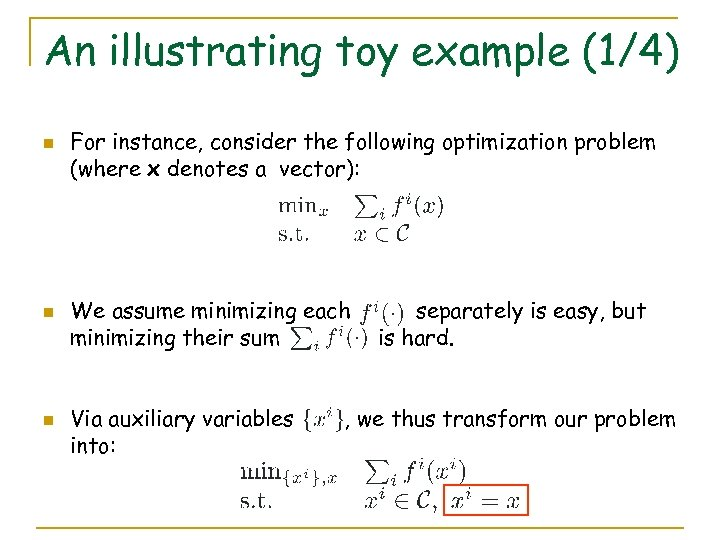An illustrating toy example (1/4) n n n For instance, consider the following optimization problem (where x denotes a vector): We assume minimizing each minimizing their sum Via auxiliary variables into: separately is easy, but is hard. , we thus transform our problem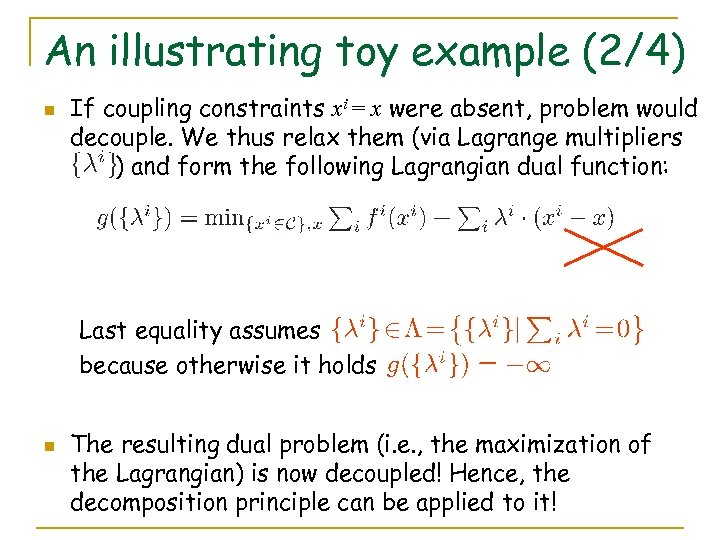An illustrating toy example (2/4) n If coupling constraints xi = x were absent, problem would decouple. We thus relax them (via Lagrange multipliers ) and form the following Lagrangian dual function: Last equality assumes because otherwise it holds n The resulting dual problem (i. e. , the maximization of the Lagrangian) is now decoupled! Hence, the decomposition principle can be applied to it!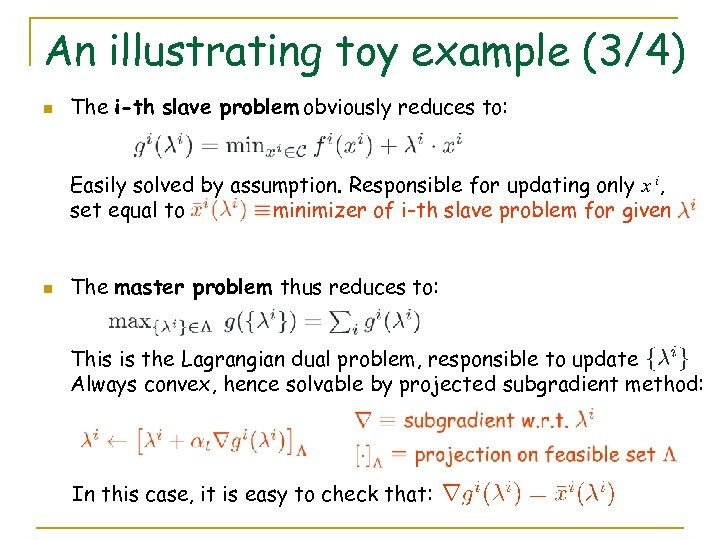An illustrating toy example (3/4) n The i-th slave problem obviously reduces to: Easily solved by assumption. Responsible for updating only x i, set equal to minimizer of i-th slave problem for given n The master problem thus reduces to: This is the Lagrangian dual problem, responsible to update Always convex, hence solvable by projected subgradient method: In this case, it is easy to check that: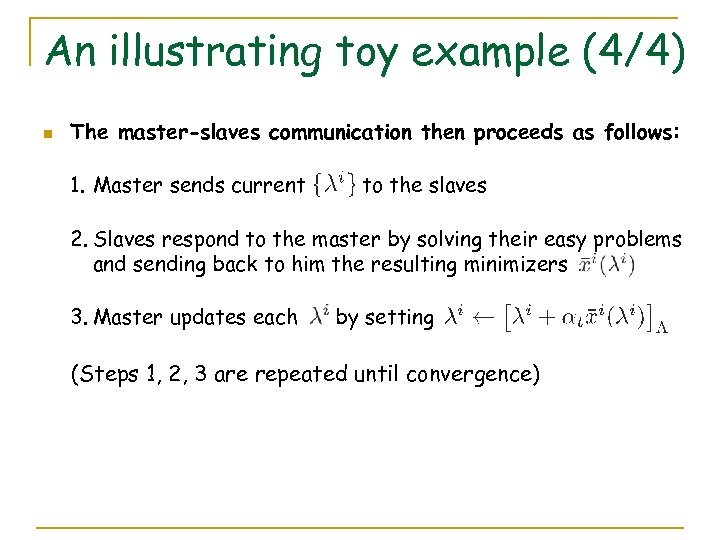An illustrating toy example (4/4) n The master-slaves communication then proceeds as follows: 1. Master sends current to the slaves 2. Slaves respond to the master by solving their easy problems and sending back to him the resulting minimizers 3. Master updates each by setting (Steps 1, 2, 3 are repeated until convergence)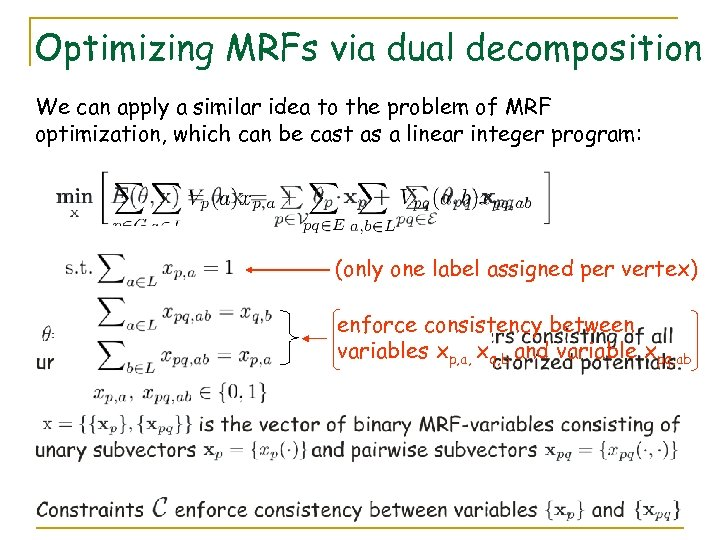Optimizing MRFs via dual decomposition We can apply a similar idea to the problem of MRF optimization, which can be cast as a linear integer program: (only one label assigned per vertex) enforce consistency between variables xp, a, xq, b and variable xpq, ab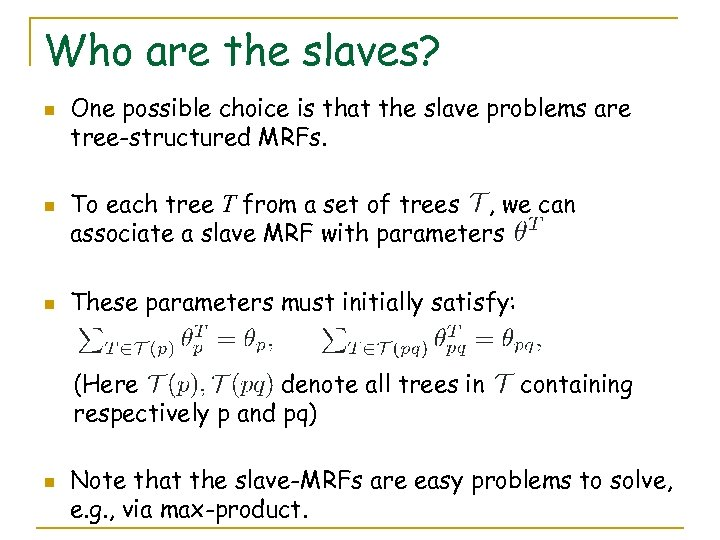Who are the slaves? n n n One possible choice is that the slave problems are tree-structured MRFs. To each tree T from a set of trees , we can associate a slave MRF with parameters These parameters must initially satisfy: (Here denote all trees in respectively p and pq) n containing Note that the slave-MRFs are easy problems to solve, e. g. , via max-product.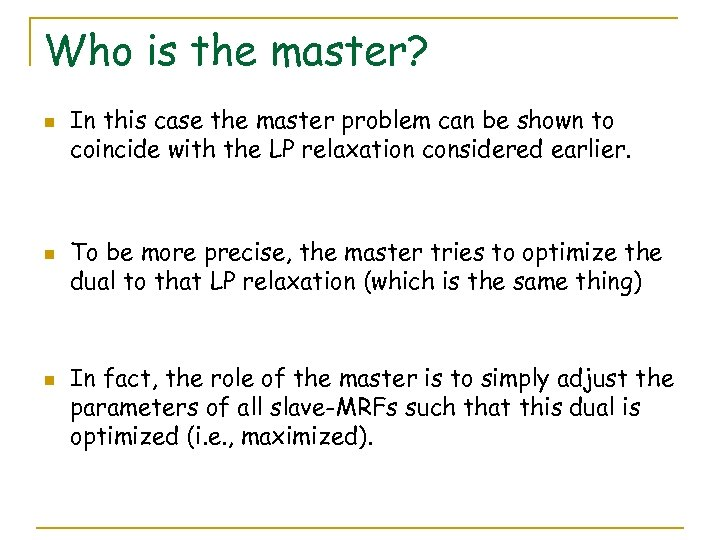Who is the master? n n n In this case the master problem can be shown to coincide with the LP relaxation considered earlier. To be more precise, the master tries to optimize the dual to that LP relaxation (which is the same thing) In fact, the role of the master is to simply adjust the parameters of all slave-MRFs such that this dual is optimized (i. e. , maximized).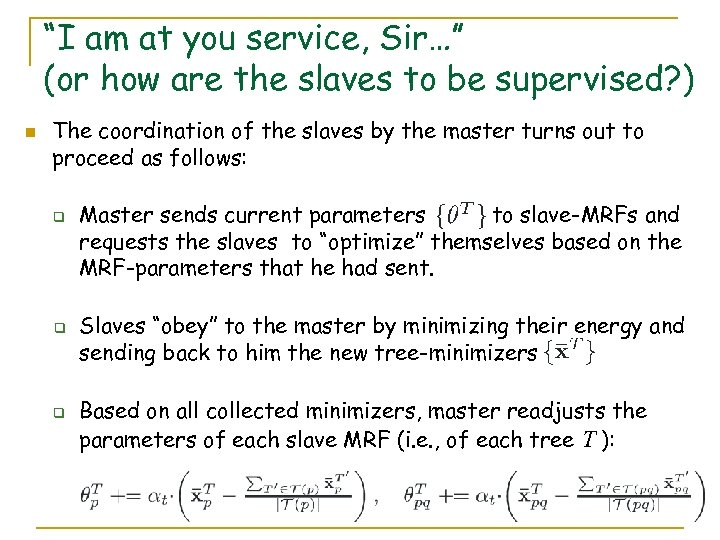“I am at you service, Sir…” (or how are the slaves to be supervised? ) n The coordination of the slaves by the master turns out to proceed as follows: q q q Master sends current parameters to slave-MRFs and requests the slaves to “optimize” themselves based on the MRF-parameters that he had sent. Slaves “obey” to the master by minimizing their energy and sending back to him the new tree-minimizers Based on all collected minimizers, master readjusts the parameters of each slave MRF (i. e. , of each tree T ):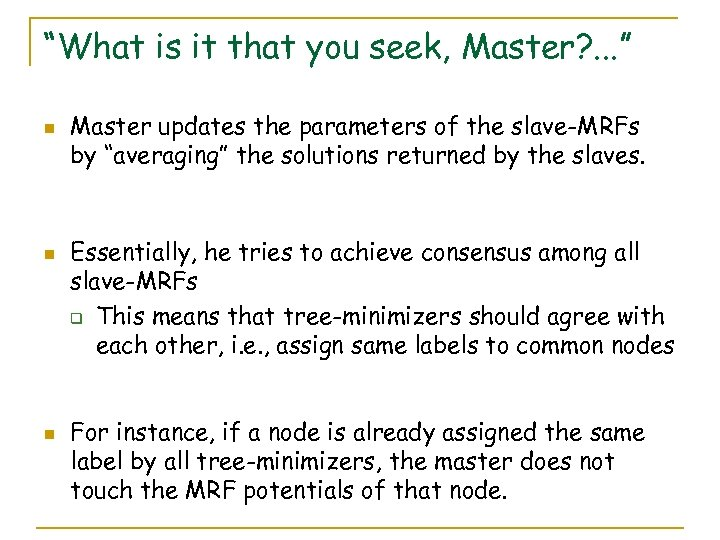“What is it that you seek, Master? . . . ” n n n Master updates the parameters of the slave-MRFs by “averaging” the solutions returned by the slaves. Essentially, he tries to achieve consensus among all slave-MRFs q This means that tree-minimizers should agree with each other, i. e. , assign same labels to common nodes For instance, if a node is already assigned the same label by all tree-minimizers, the master does not touch the MRF potentials of that node.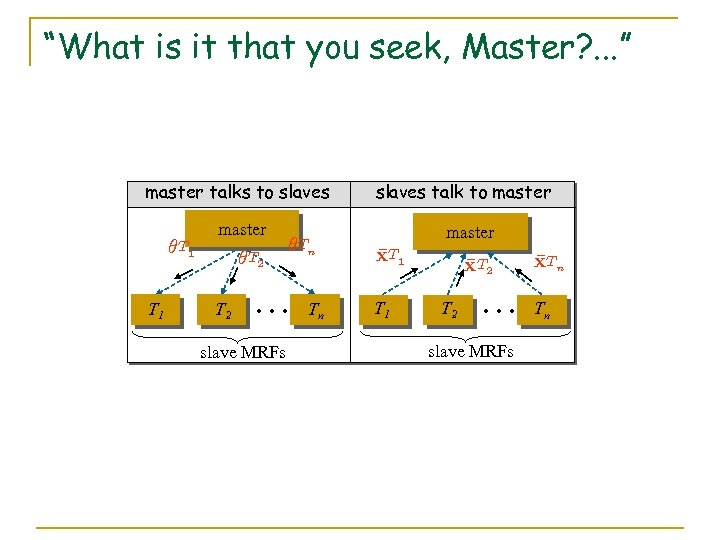“What is it that you seek, Master? . . . ” master talks to slaves µ T 1 master µ T 2 µ Tn … slave MRFs Tn slaves talk to master x. T 1 ¹ T 1 x. T 2 ¹ T 2 … slave MRFs x. Tn ¹ Tn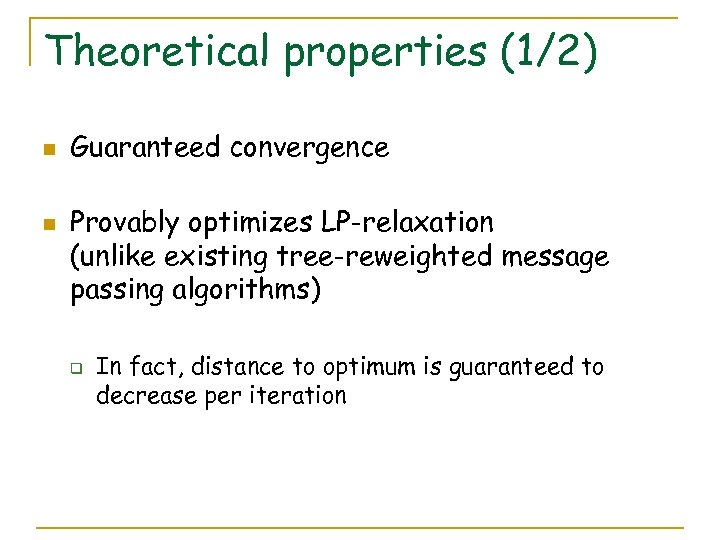Theoretical properties (1/2) n n Guaranteed convergence Provably optimizes LP-relaxation (unlike existing tree-reweighted message passing algorithms) q In fact, distance to optimum is guaranteed to decrease per iteration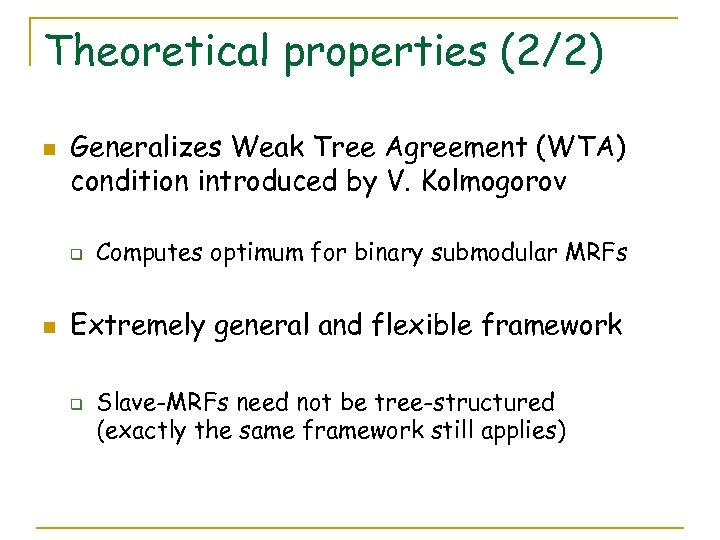Theoretical properties (2/2) n Generalizes Weak Tree Agreement (WTA) condition introduced by V. Kolmogorov q n Computes optimum for binary submodular MRFs Extremely general and flexible framework q Slave-MRFs need not be tree-structured (exactly the same framework still applies)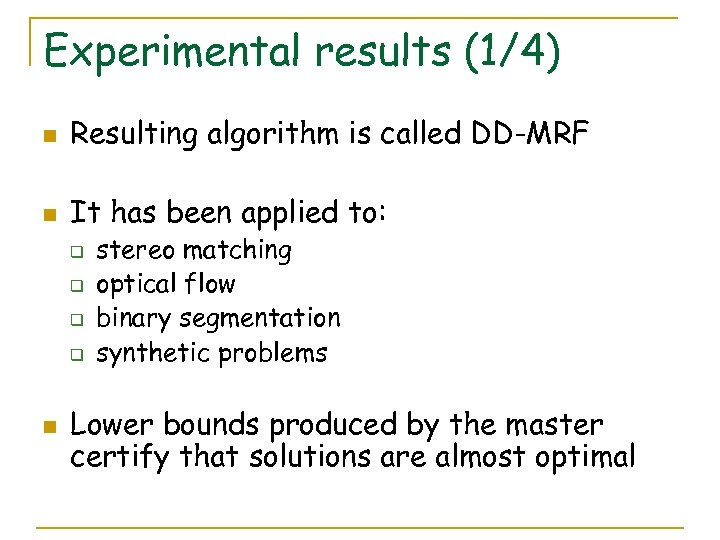Experimental results (1/4) n Resulting algorithm is called DD-MRF n It has been applied to: q q n stereo matching optical flow binary segmentation synthetic problems Lower bounds produced by the master certify that solutions are almost optimal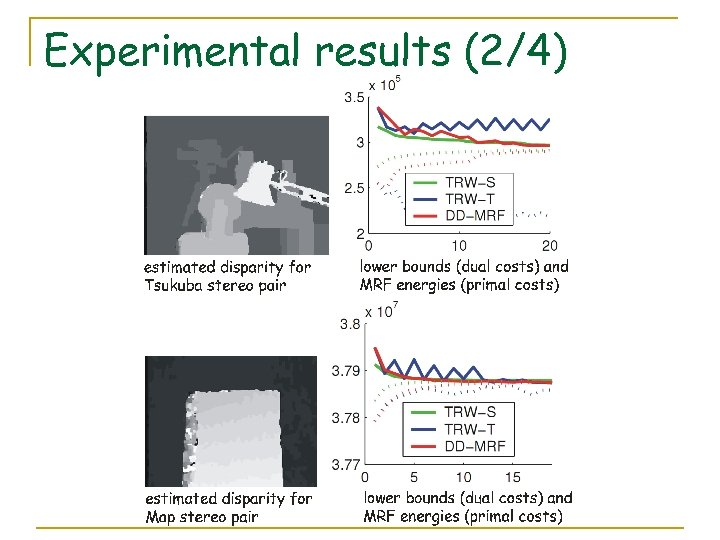Experimental results (2/4)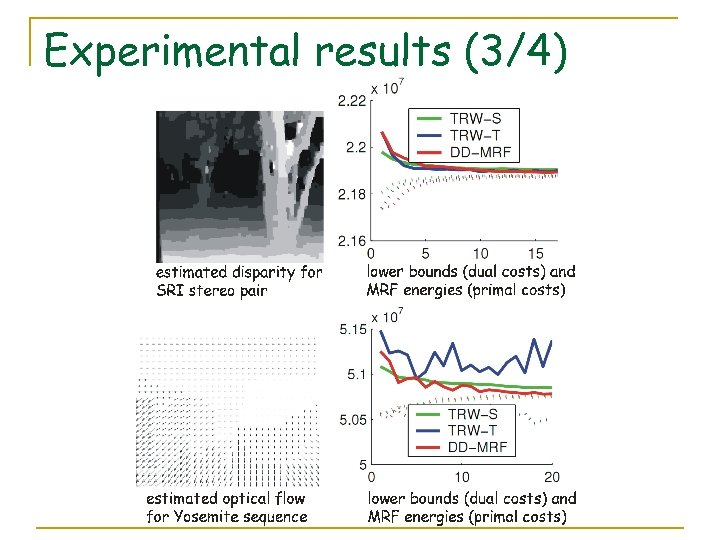Experimental results (3/4)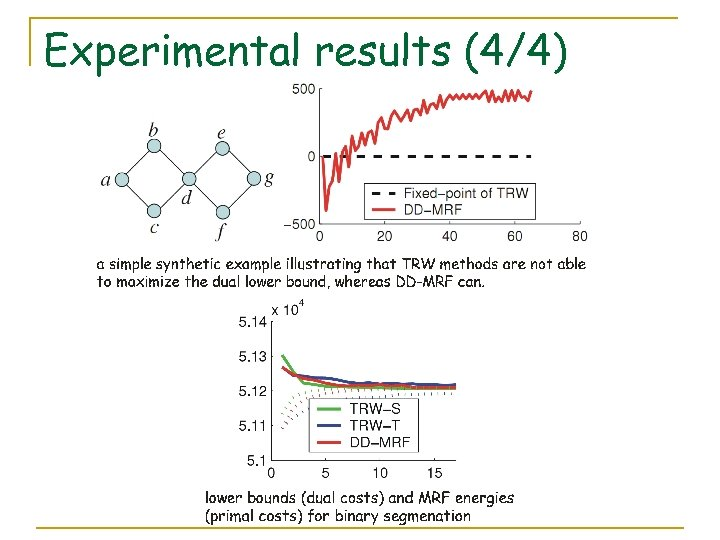Experimental results (4/4)Take home messages 1. Relaxing is always a good idea (just don’t overdo it!) 2. Take advantage of duality, whenever you can Thank you!